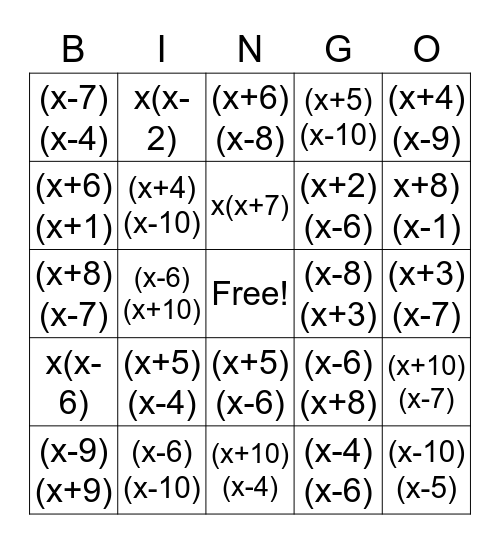# Factor BingoThis bingo card has a free space and 24 words: (x+5)(x-4), (x-6)(x-10), x(x+7), (x-9)(x+9), (x+4)(x-9), (x-7)(x-4), (x+6)(x+1), x(x-2), (x-8)(x+3), (x+5)(x-6), (x+2)(x-6), (x+8)(x-7), (x-6)(x+8), (x+10)(x-7), (x+4)(x-10), (x-4)(x-6), (x+3)(x-7), (x+6)(x-8), x+8)(x-1), (x-6)(x+10), (x-10)(x-5), x(x-6), (x+5)(x-10) and (x+10)(x-4).

## Play Online